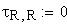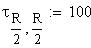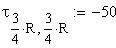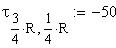Heat Flow on a Square PlateThis template can be used to solve for the steady-state temperature distribution of a square plate, using the partial differential equation solver multigrid.
Find the temperature T(x,y) of a square plate with a constant internal heat source. The boundary of the source is pinned at zero degrees.
At any point free of the heat source, the heat equation reduces to Laplace's equation in two variables: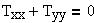Size of the grid is (R + 1) by (R + 1):Sets the dimensions of the source r: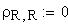Position and strength of source:Contour plot of temperature (showing lines of constant temperature).
Surface plot of temperature
A different configuration of heat sources (3 hot spots and one cold) gives the following: